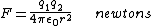# permittivity

(redirected from Permettivity)
Also found in: Dictionary.

## Permittivity

A property of a dielectric medium that determines the forces that electric charges placed in the medium exert on each other. If two charges of q1 and q2 coulombs in free space are separated by a distance r meters, the electrostatic force F newtons acting upon each of them is proportional to the product of the charges and inversely proportional to the square of the distance between them. Thus, F is given by Eq. (1),

(1)where 1/(4&pgr;ε0) is the constant of proportionality, having the magnitude and dimensions necessary to satisfy Eq. (1). This condition leads to a value for ε0, termed the permittivity of free space, given by Eq. (2), where
(2)c is the velocity of light in vacuum.

If now the charges are placed in a dielectric medium that is homogeneous and isotropic, the force on each of them is reduced by a factor εr, where εr is greater than 1. This dimensionless scalar quantity is termed the relative permittivity of the medium, and the product ε0εr is termed the absolute permittivity ε of the medium.

A consequence is that if two equal charges of opposite sign are placed on two separate conductors, then the potential difference between the conductors will be reduced by a factor εr when the conductors are immersed in a dielectric medium compared to the potential difference when they are in vacuum. Hence a capacitor filled with a dielectric material has a capacitance εr times greater than a capacitor with the same electrodes in vacuum would have. Except for exceedingly high applied fields, unlikely normally to be reached, εr is independent of the magnitude of the applied electric field for all dielectric materials used in practice, excluding ferroelectrics. See Capacitance, Capacitor, Ferroelectrics

## permittivity

[‚pər·mə′tiv·əd·ē]
(electricity)
The dielectric constant multiplied by the permittivity of empty space, where the permittivity of empty space (ε0) is a constant appearing in Coulomb's law, having the value of 1 in centimeter-gram-second electrostatic units, and of 8.854 × 10-12 farad/meter in rationalized meter-kilogram-second units. Symbolized ε.
Site: Follow: Share:
Open / Close